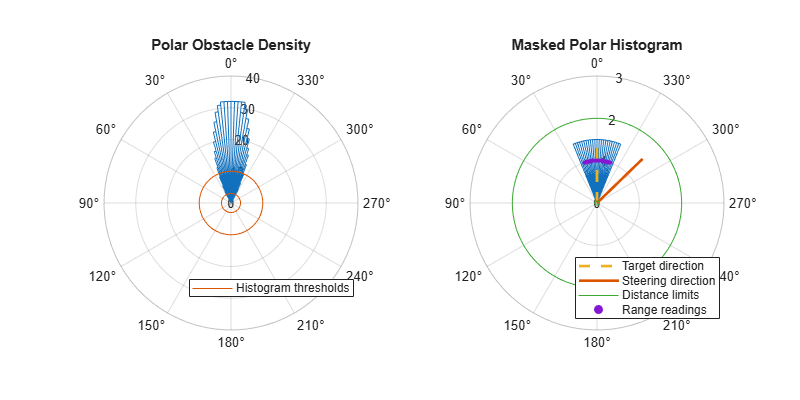# show

Display `VectorFieldHistogram` information in figure window

## Syntax

``show(vfh)``
``show(vfh,'Parent',parent)``
``h = show(___)``

## Description

example

````show(vfh)` shows histograms calculated by the VFH+ algorithm in a figure window. The figure also includes the parameters of the `controllerVFH` object and range values from the last object call.```
````show(vfh,'Parent',parent)` sets the specified axes handle, `parent`, to the axes.```
````h = show(___)` returns the figure object handle created by `show` using any of the arguments from the previous syntaxes.```

## Examples

collapse all

This example shows how to calculate a steering direction based on input laser scan data.

Create a `controllerVFH` object. Set the `UseLidarScan` property to `true`.

```vfh = controllerVFH; vfh.UseLidarScan = true;```

Input laser scan data and target direction.

```ranges = 10*ones(1,500); ranges(1,225:275) = 1.0; angles = linspace(-pi,pi,500); targetDir = 0;```

Create a `lidarScan` object by specifying the ranges and angles.

`scan = lidarScan(ranges,angles);`

Compute an obstacle-free steering direction.

`steeringDir = vfh(scan,targetDir);`

Visualize the `VectorFieldHistogram` computation.

```h = figure; set(h,'Position',[50 50 800 400]) show(vfh)```## Input Arguments

collapse all

Vector field histogram algorithm, specified as a `controllerVFH` object. This object contains all the parameters for tuning the VFH+ algorithm.

Axes properties, specified as a handle.

## Output Arguments

collapse all

Axes handles for VFH algorithm display, specified as an `Axes` array. The VFH histogram and `HistogramThresholds` are shown in the first axes. The binary histogram, range sensor readings, target direction, and steering directions are shown in the second axes.